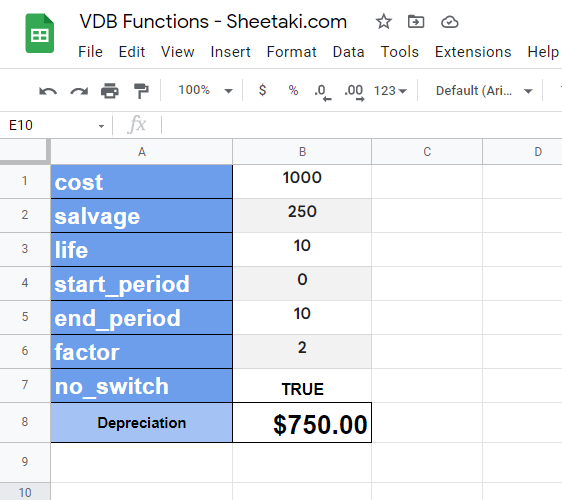# How to Use VDB Function in Google Sheets

The VDB function in Google Sheets is a useful function when you need to return the depreciation of a given asset for a particular period.

By default, this function uses the double-declining balance method, which is a form of accelerated depreciation.

The rules for using the VDB function in Google Sheets are as follows:

• The function requires several arguments to describe the asset (cost, salvage, life ) and the periods of depreciation to calculate (start_period and end_period)
• We can also specify the rate of balance decline and whether we can switch to a straight-line depreciation when necessary.
• Using these arguments, the function then outputs the depreciation of the asset over the specified period.

Let’s begin with a quick use-case of this function.

You have an asset worth \$500 that has a useful life of 10 years. At the end of its lifespan, it’s worth only \$250. Given a rate of decline of 2, how much does the asset depreciate each year?

With the `VDB` function it becomes an easy calculation. We already have the initial cost (\$500), the salvage price (\$250), and lifespan (10 years or periods). To get the depreciation per year, we just need to adjust the start_period and end period for each corresponding year.

Now that we’ve seen a possible use-case for this function, let’s learn how to write the `VDB` function ourselves in Google Sheets. We’ll later test out the function with real values to see the function in action.

## The Anatomy of the VDB Function

So the syntax (the way we write it) of the `VDB` function in Google Sheets is as follows:

```=VDB(cost, salvage, life, start_period, end_period, [factor], [no_switch])
```

Let’s dissect this function and understand what each of these arguments means:

• = the equal sign is how we start any function in Google Sheets.
• VDB() is our `VDB` function. It calculates the depreciation of an asset over a particular period.
• cost refers to the initial cost of the asset.
• salvage refers to the salvage value or the value of the given asset at the end of its lifetime.
• life refers to the number of periods of depreciation or how long the asset is useful for.
• start_period is the starting period of our depreciation calculation.
• end_period is the ending period of our depreciation calculation.
• factor refers to the rate of balance decline; by default, it is set to 2.
• no_switch is an optional argument that allows us to switch to a straight-line depreciation if the depreciation ends up being greater than the declining balance calculation.

## A Real Example of Using VDB Function

Let’s look at a specific example of the `VDB` function being used in a Google Sheets spreadsheet.

In the example below, we have a table of our `VDB` function arguments in order. We can easily plug in our values and get the result in cell B9.To get the value in cell B9, we just need to use the following formula:

```=VDB(B1, B2, B3, B4, B5, B6, B7)
```

You can make a copy of the spreadsheet above using the link I have attached below.

In the example below, we’ve extended the worksheet further by adding in computation for the asset’s actual value and adding a chart.If you’re ready to try out the `VDB` function in Google Sheets, let’s begin writing it ourselves!

## How to Use VDB Function in Google Sheets

1. In this example, we’ll be recreating the depreciation calculator shown above. First, we need to set up our table of arguments, as seen below.2. Next, we must select where to put the result of our function. In this example, we’ll choose cell B8.3. Afterward, we can start our formula by typing the equal sign ‘=‘, followed by ‘VDB(‘.
4. A tooltip pop-up may appear with information on the `VDB` function. We can click on the arrow on the top-right-hand corner of the box to hide it from view.5. The next step involves typing in our arguments in the Formula bar. Afterward, simply hit Enter on your keyboard to let the function return the final result.6. As seen below, we can plug in different values to our worksheet and obtain different results. In the picture below, we can see that the calculator returns the depreciation from the starting period to the final period.You now know everything there is to know about using the `VDB` function in Google Sheets.  Hopefully, this step-by-step guide shows how easy it is to perform calculations involving depreciation of assets.

You can now use the `VDB` function in Google Sheets together with the various other Google Sheets formulas available to create spreadsheets that solve any use-case.Our goal this year is to create lots of rich, bite-sized tutorials for Google Sheets users like you. If you liked this one, you'd love what we are working on! Readers receive ✨ early access ✨ to new content.

##### You May Also Like## How to Make a Scatter Plot in Google Sheets

Google Sheets has a useful feature that allows you to make scatter plot charts from your dataset. Scatter…## How to Use EQ Function in Google Sheets

The EQ function in Google Sheets is used to compare two specified values, and returns the value ‘TRUE’…## How to Use SORT Function in Google Sheets

The SORT function in Google Sheets is useful to sort and return the rows of a range by the…## How to Use TIMEVALUE Function in Google Sheets

The TIMEVALUE function in Google Sheets is useful if you want to give a numerical value to a…